Courses

# Engineering Mathematics 9

## 10 Questions MCQ Test RRB JE for Computer Science Engineering | Engineering Mathematics 9

Description
This mock test of Engineering Mathematics 9 for Computer Science Engineering (CSE) helps you for every Computer Science Engineering (CSE) entrance exam. This contains 10 Multiple Choice Questions for Computer Science Engineering (CSE) Engineering Mathematics 9 (mcq) to study with solutions a complete question bank. The solved questions answers in this Engineering Mathematics 9 quiz give you a good mix of easy questions and tough questions. Computer Science Engineering (CSE) students definitely take this Engineering Mathematics 9 exercise for a better result in the exam. You can find other Engineering Mathematics 9 extra questions, long questions & short questions for Computer Science Engineering (CSE) on EduRev as well by searching above.
QUESTION: 1

### A sample of 15 data is as follows: 17, 18, 17, 17, 13, 18, 5, 5, 6, 7, 8, 9, 20, 17, 3. the mode of the data is

Solution:

Mode refers the most frequently appeared value (which is 17 in this case).

QUESTION: 2

### In a Poisson distribution if 2P(X = 1) = P(X = 2), then what is the mean?

Solution:

If X is random variable, then Poisson’s distribution is given by: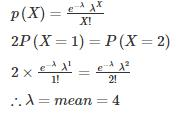QUESTION: 3

### The continuous distribution variable X is distributed uniformly where X ~ U(6,12). Find the mean of X?

Solution:

X ~ U(6,12)

Comparing with X ~ U(a,b)

∴ a = 6 and b = 12
Mean of uniform distributed variable is given as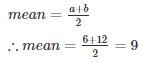QUESTION: 4

Consider a discrete random variable Y that takes values -2, -1, 0, 1, and 2 with probability 0.2 each. The values of the cumulative distribution function G(y) at y = -1 and y = 1 are _____ and _____ respectively.

Solution: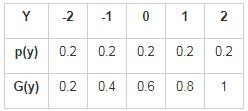G(-1) = p(-2) + p(-1)

= 0.2 + 0.2 = 0.4

G(1) = p(-2) + p(-1) + p(0) + p(1)

= 0.2 + 0.2 + 0.2 + 0.2

= 0.8

QUESTION: 5

A die is tossed thrice. Success is getting 2 or 5 on a toss. What is the variance of the number of success?

Solution:

P(success) = Probability of getting 2 or 5 is 2/6 = 1/3

P(failure) = Probability of not getting 2 or 5 is 4/6 = 2/3
Probability of 0 success and 3 failure is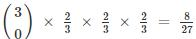Probability of 1 success and 2 failure is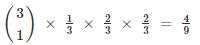Probability of 2 success and 1 failure is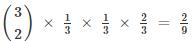Probability of 3 success and 0 failure is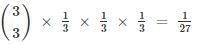x is the number of success and p(x) is probability of x times success.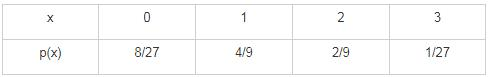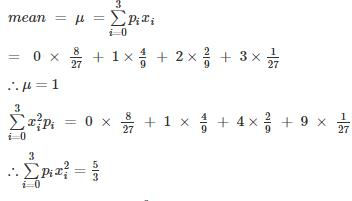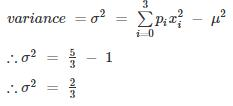QUESTION: 6

What is the variance of the random variable X whose probability mass function is given below?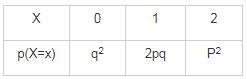Solution:

q2 + 2pq + p2 = 1

(p+q)2 = 1

∴ (p+q) = 1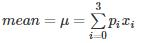= 0 × q2 + 1 × 2pq + 2 × p2

= 2p (q+p)

∴ μ = 2p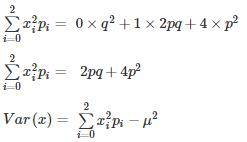Var(x) = 2pq + 4p2 − 4p2

Var(x) = 2pq

QUESTION: 7

3, k, 2, 8, m, 3

The arithmetic mean of the list of numbers above is 4. If k and m are integers and k ≠ m, what is the median of the list?

Solution:

Mean = 4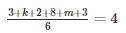k ≠ m so k = m = 4 is out.

{k, m} = {1, 7} or {2, 6} or {3, 5}

for median of {3, k, 2, 8, m, 3}

{1, 2, 3, 3, 7, 8} or {2, 2, 3, 3, 6, 8} or {2, 3, 3, 3, 5, 8}

Median: (3 + 3) /2 = 3

*Answer can only contain numeric values
QUESTION: 8

If a random variable X has a Poisson distribution with variance 6, then expectation [E(X + 3)2] equals _____.

Solution:

Var(X) = E(X) = 6

Var(X) = E(X2) - (E(X))2

6 = E(X2) – 36

∴ E(X2) = 42

[E(X + 3)2]

= E(X2 + 6X + 9 )

= E(X2) + 6E(X) + 9

= 42 + 6× 6 + 9

= 87

QUESTION: 9

The probability density function of a random variable function y has the following probability function: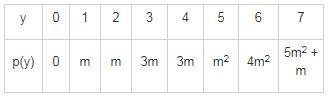find p(y ≥ 5)?

Solution:

Since y is a random variable: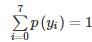= 0 + m+ m + 3m + 3m + m2 + 4m2 + 5m2 + m = 1

10m2 + 9m−1 = 0

(m+1) (10m−1) = 0

∴ m = −1or m = 1/10
since probability cannot be in negative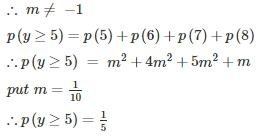QUESTION: 10

In a binomial distribution, the mean is 4 and variance is 3. Then the mode is

Solution:

np = 4 and npq = 3

thus q = (3/4) and p = (1-q) = (1/4)

mode is an integer such that np+p > x > np-q

⇒ 4 +(1/4) > x >4-(3/4)

⇒ (13/4)<x<(17/4)

3.25<x<4.25

⇒ x = 4